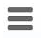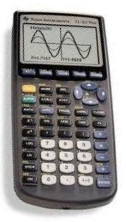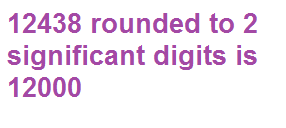﻿ Significant Figures (Sig Fig) Rounding Calculator ﻿# Significant Figures (Sig Fig) Rounding CalculatorEnter a Number:

How Many Significant Digits To Round To:

﻿

This Significant Figures Rounding Calculator rounds a given number to the amount of significant digits that you specify.

This rounding number which you specify cannot be a negative number and it must be greater than 0. A number with 0 significant digits would be 0. So the number to round to must be a positive. Also, the rounding number specified must be a number that is either equal to or less than the amount of significant digits present in the number. If a number only has 2 significant digits in it and you specify that you want it rounded to 5, for example, this is an impossibility. If a number only has 2 significant digits, the maximum significant digits it can be rounded to is 2 significant digits. A rounding number greater than 2 would make the end result falsely more accurate than it is, which would be a false representation. So this calculator only allows a rounding number equal to or less than the amount of significant digits in the number, or else it will throw an error, warning you of this.

Significant figures, or digits, are the values in a number that can be counted on to be accurate. Significant digits in a number are those values which can be known with certainty or a high degree of confidence, while insignificant digits are those which we do not trust as very accurate.

Significant digits are used extensively during measurements. Different measurement tools can record measurements of differing accuracy. Some measurement tools can record much more in detail than other measuring tools. For example, if we have a ruler that only measures centimeters, we can measure to one-hundredth of a meter. If we now change the ruler and get one which measures millimeters, we can measure to one-thousandth of a meter. Thus, we can have an extra significant digit, because the ruler is more detailed and allows for more accuracy of measurement.

It is important to be honest when making a measurement, so that the resulant value does not appear to be more accurate than the equipment used to make the measurement allows. And how we make the recorded value honest is by controlling the number of digits, or significant figures, used to report the measurement. The recorded value cannot have more significant digits than the measuring tool allows. This is why using the proper amount of significant digits is so important.

To use this calculator, a user simply enters in a number into the first text box and then the number of significant digits s/he would like to round that number to in the second text box, and clicks the 'Calculate' button. The resultant value will be the number entered rounded to the number of significant digits desired.

Being that electronics, like any other science, deals with measurements, knowing how to round to a given number of significant figures may be important. Depending on the measuring tool in use determines how accurate it can measure. Using the proper number of significant figures may be extremely important.

Examples

What is 12300 roundeed to 2 significant digits?

12000

The original number has 3 significant digits. Rounding it to 2 significant digits makes it 12000.

What is 15875 rounded to 4 significant digits?

15880

The original number has 5 signifiant digits. Rounding it to 4 significant digits makes it 15880.

What is 0.000375 rounded to 2 significant digts?

0.00038

The original number has 3 significant digits. Rounding it to 2 significant digits m akes it 0.00038.

Related Resources

﻿